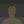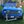0%
0%

# Super custom PWM - FPGA

Make a FPGA with lots of PWM ports, all of them 32 bit, and easy to program!

Similar projects worth following
262 views
To learn more about FPGAs, I created an FPGA that can generate a whole bunch of 32-bit PWM ports. This first version has 8 PWMs, each running 32 bit.

Please see my "first project" on how to setup everything:

I used an Intel MAX10 FPGA (10M08SAE144C8G), and then a ATMEGA256RFR2 to interface with the FPGA over SPI.  The ATMEGA256RFR2 is 3.3 V, so compatible with the FPGA.

For this project, I created a SPI interface on the FPGA.  The ATMEGA256RFR2 is the master.  Since this is my FPGA, and maybe we could move away from the "slave" term, I called the FPGA the "sidekick".

Anyway, I also added a "register map" to the FPGA.  This version has 8 indepedent PWMs, all running at 32 bit, and 50 MHz.  I tried to comment the heck out of the code.  This is a complete project, so future versions could have more features.

Enjoy!

The PWM period can be from 50 ns all the way up to ~86 seconds.  The PWM on time can be as short as 25 ns.  Not bad!  :)

Below is the teraterm session between the PC and the ATMEGA256RFR2.  Register (decimal) 198 is the SPI register for output (i.e., UDR0).  Not show, I set the chip select pin to 0 (from 1).  Then, I enter 1 to edit a register.  Then, address "15".  Next, I set the 32 bit register to 2 (i.e. the period of the PWM is 2 ticks of the 50 MHz FPGA clock).  Then, I write 1 to register 16 (i.e, for the PWM to be high for 1 tick of the 50 MHz FPGA).  I highlighted the session in orange...

This gave rise to the super short PWM output on pin 41 of the FPGA (i.e., one tick of the 50 MHz clock, and a period of two ticks of the 50 MHz clock):

Next, I programmed in larger values for the PWM settings (i.e., the programmed values for the 32 bit register were decimal 1, 0, 0, 0 which will mean an actual decimal value of 16777216 ticks for the PWM output to be high, or 336 ms high), and a period of 33554432 ticks (or 671 ms).

And voila!

For interest, here is the Quartus report:

Flow Status Successful - Sun Nov 01 22:36:01 2020
Quartus Prime Version 20.1.0 Build 711 06/05/2020 SJ Lite Edition
Revision Name spi_pwm_micro_test
Top-level Entity Name spi_pwm_micro_test
Family MAX 10
Device 10M08SAE144C8G
Timing Models Final
Total logic elements 1,628 / 8,064 ( 20 % )
Total registers 923
Total pins 18 / 101 ( 18 % )
Total virtual pins 0
Total memory bits 0 / 387,072 ( 0 % )
Embedded Multiplier 9-bit elements 0 / 48 ( 0 % )
Total PLLs 0 / 1 ( 0 % )
UFM blocks 0 / 1 ( 0 % )
ADC blocks 0 / 1 ( 0 % )

• ### Main verilog HDL code

```// Main module - the ports for the FPGA, register map handling
module spi_pwm_micro_test(
input wire clk, // 50 MHz clock, pin 27
input wire bar_ss, // SPI chip select, pin 93
input wire mosi, // SPI master output, pin 92
input wire sck, // SPI clock, pin 99
output wire miso, // SPI sidekick output, pin 101
output wire led1, // LED1 pin 132
output wire led2, // LED2 pin 134
output wire led3, // LED3 pin 135
output wire led4, // LED4 pin 140
output wire led5, // LED5 pin 141
output wire pwm_wire0, // PIN 60
output wire pwm_wire1, // PIN 59
output wire pwm_wire2, // PIN 56
output wire pwm_wire3, // PIN 55
output wire pwm_wire4, // PIN 47
output wire pwm_wire5, // PIN 46
output wire pwm_wire6, // PIN 43
output wire pwm_wire7); // PIN 41

//////
// Register map
reg [31:0] max_count_0;
reg [31:0] compare_val_0;
reg [31:0] max_count_1;
reg [31:0] compare_val_1;
reg [31:0] max_count_2;
reg [31:0] compare_val_2;
reg [31:0] max_count_3;
reg [31:0] compare_val_3;
reg [31:0] max_count_4;
reg [31:0] compare_val_4;
reg [31:0] max_count_5;
reg [31:0] compare_val_5;
reg [31:0] max_count_6;
reg [31:0] compare_val_6;
reg [31:0] max_count_7;
reg [31:0] compare_val_7;

// Initial values for the register map
initial begin
max_count_0 <= 32'h02FAF080;
compare_val_0 <= 32'h017D7840;

max_count_1 <= 32'h02FAF080;
compare_val_1 <= 32'h017D7840;

max_count_2 <= 32'h02FAF080;
compare_val_2 <= 32'h017D7840;

max_count_3 <= 32'h02FAF080;
compare_val_3 <= 32'h017D7840;

max_count_4 <= 32'h02FAF080;
compare_val_4 <= 32'h017D7840;

max_count_5 <= 32'h02FAF080;
compare_val_5 <= 32'h017D7840;

max_count_6 <= 32'h02FAF080;
compare_val_6 <= 32'h017D7840;

max_count_7 <= 32'h02FAF080;
compare_val_7 <= 32'h017D7840;

end

//////
// Registers for the SPI interface
wire [31:0] spi_write_data;

initial begin
end

// SPI module

// Register map in/out
always @(negedge clk) begin
// Writing registers
8'd1 : max_count_0 <= spi_write_data;
8'd2 : compare_val_0 <= spi_write_data;
8'd3 : max_count_1 <= spi_write_data;
8'd4 : compare_val_1 <= spi_write_data;
8'd5 : max_count_2 <= spi_write_data;
8'd6 : compare_val_2 <= spi_write_data;
8'd7 : max_count_3 <= spi_write_data;
8'd8 : compare_val_3 <= spi_write_data;
8'd9 : max_count_4 <= spi_write_data;
8'd10 : compare_val_4 <= spi_write_data;
8'd11 : max_count_5 <= spi_write_data;
8'd12 : compare_val_5 <= spi_write_data;
8'd13 : max_count_6 <= spi_write_data;
8'd14 : compare_val_6 <= spi_write_data;
8'd15 : max_count_7 <= spi_write_data;
8'd16 : compare_val_7 <= spi_write_data;
endcase

endcase

end
end

//////
// PWM modules
pwm_module pwm0(clk, max_count_0, compare_val_0, pwm_wire0);
pwm_module pwm1(clk, max_count_1, compare_val_1, pwm_wire1);
pwm_module pwm2(clk,...```

• ### SPI interface verilog HDL code

```// The SPI interface
// Supports loop-back for BER testing
// 32 bit register values
module spi_interface(
input wire clk,
input wire bar_ss,
input wire mosi,
input wire sck,
output wire miso,
output wire [31:0] spi_write_data,
);

//////
// Observe the serial clock
reg [2:0] sck_r;
initial begin
sck_r <= 3'b000;
end
always @ (posedge clk) begin
sck_r <= {sck_r[1:0], sck};
end

// Detect the rising edge
wire sck_risingedge = (sck_r[2:1]==2'b01);
// Detect the falling edge
wire sck_fallingedge = (sck_r[2:1]==2'b10);

//////
// Observe the mosi wire
reg [1:0] mosi_r;

always @ (posedge clk) begin
mosi_r <= {mosi_r, mosi};
end
// The mosi data
wire mosi_data = mosi_r;

//////
// Observe bar_ss
reg[2:0] bar_ss_r;
initial begin
bar_ss_r <= 3'b111;
end

always @ (posedge clk) begin
bar_ss_r <= {bar_ss_r[1:0], bar_ss};
end

// Detect the falling edge
wire bar_ss_fallingedge = (bar_ss_r[2:1]==2'b10);
// Observation of bar_ss
wire bar_ss_observe = bar_ss_r;

//////
// Place to store input data
reg [5:0] bit_count;
reg [31:0] data_rx;
reg [31:0] data_tx;

initial begin
bit_count <= 6'd0;
data_tx <= 32'd0;
end

// Output is tristate, until bar_ss_observe becomes 0
assign miso = (!bar_ss_observe) ? data_tx : 1'bz;

// Place to store the state variable
// 0 Command arriving
// 2 Read register, get the register value
// 3 Read register, sending bits 31:0
// 8 Write register, address arriving
// 9 Write register, bits 30:0 arriving
// 10 Write register, bit 31 is ready, write the register
reg [3:0] spi_state;
initial begin
spi_state <= 4'd0;
end

reg [31:0] spi_write_data_reg;
initial begin
spi_write_data_reg <= 32'd0;
end

// On the rising edge of the serial clock, data is ready for processing
always @(posedge clk) begin

if (bar_ss_fallingedge == 1'b1) begin
// On the falling edge of bar_ss, clear bit count and set spi state
bit_count <= 6'd0;
spi_state <= 4'd0;

end else if ((bar_ss_observe == 1'b0) && (sck_risingedge == 1'b1)) begin

// If we have 7 bits, figure out the next state from the input bit
if (bit_count == 6'd7) begin
if ({data_rx[6:0], mosi_data} == 8'd0) begin
spi_state <= 4'd1;
end else if ({data_rx[6:0], mosi_data} == 8'd1) begin
// the master is asking to write a register
spi_state <= 4'd8;
end
end

if (bit_count == 6'd15) begin

// If we are doing a SPI read, and are up to 15 bits total, prepare the register address, ready to send the register back
if (spi_state == 4'd1) begin
spi_state <= 4'd2;

end else if (spi_state == 4'd8) begin
// If we are doing a SPI write, and are up to 15 bits total, prepare the register address, ready to recieve the next 32 bits for the register
spi_state <= 4'd9;

end
end

// Have 31 bits for the register write, so get the mosi data to make 32 bits, set the state to 10
if ((bit_count == 6'd47) && (spi_state == 4'd9)) begin
spi_write_data_reg <= {data_rx[30:0], mosi_data};
spi_state <= 4'd10;
end

// Keep incrementing and sampling bits
bit_count <= bit_count + 6'd1;
data_rx <= {data_rx[30:0], mosi_data};

end else if ((bar_ss_observe == 1'b0) && (sck_fallingedge == 1'b1)) begin

// Transfer the register value to the local register, and change the state
if (spi_state == 4'd2) begin
spi_state <= 4'd3;

end else if (spi_state == 4'd10) begin
spi_state <= 4'd0;
bit_count <= 6'd0;

end else begin

// Near the end of register read, the next 8 bits will be a new command
if ((spi_state...```

• ### PWM module verilog HDL code

// PWM module
// inputs
//    clk - the clock signal, will use posedge
//    max_count - the highest count value of the counter
//    compare_val - the comparison value for the counter
// outputs
//    cmp - compares the internal counter to compare_val
//
// note
//    a counter that always counts up to max_count
//    compares the counter to compare_val - if the counter is lower, output 1, otherwise output 0

module pwm_module(
input wire clk,
input wire [31:0] max_count,
input wire [31:0] compare_val,
output wire cmp
);

// Internal counter
reg [31:0] cnt;

// Set to 0 at start
initial begin
cnt <= 32'h00000000;
end

always @(posedge clk) begin

if (cnt < max_count) begin
// If the counter is less than max_count, increment
cnt <= cnt + 32'h00000001;
end else begin
// otherwise, set to 0
cnt <= 0;
end
end

// When count is less than the compare value, output 1, otherwise 0
assign cmp = (cnt < compare_val) ? 1'b1 : 1'b0;

endmodule

• ### SDC file

# inform Quartus that the clk port brings a 50MHz clock into our design so
# that timing closure on our design can be analyzed
create_clock -name clk -period "50MHz" [get_ports clk]
# inform Quartus that the LED output port has no critical timing requirements
# it’s a single output port driving an LED, there are no timing relationships
# that are critical for this
set_false_path -from * -to [get_ports led1]
set_false_path -from * -to [get_ports led2]
set_false_path -from * -to [get_ports led3]
set_false_path -from * -to [get_ports led4]
set_false_path -from * -to [get_ports led5]

Share

## Similar Projects

Project Owner Contributor

### Philips LivingColors gen1 WiFi addonStefkuhb

Project Owner Contributor

### usb oscilloscope data loggingBDM

# Does this project spark your interest?

Become a member to follow this project and never miss any updates